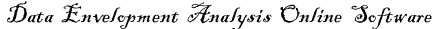# linear programming

In mathematics, linear programming (LP) problems involve the optimization of a linear objective function, subject to linear equality and inequality constraints which all would be inrtoduced in the following example.

Put very informally, LP is about trying to get the best outcome (e.g. maximum profit, least effort, etc) given some list of constraints (e.g. only working 30 hours a week, not doing anything illegal, etc), using a linear mathematical model.

More formally, given a polytope (for example, a polygon or a polyhedron), and a real-valued function$f(x_1, x_2, \dots, x_n)=a_1x_1+a_2x_2+\cdots +a_nx_n+b\,$

defined on this polytope, the goal is to find a point in the polytope where this function has the smallest (or largest) value. Such points may not exist, but if they do, searching through the polytope vertices is guaranteed to find at least one of them.

Linear programs are problems that can be expressed in canonical form:

Maximize$\mathbf{c}^T \mathbf{x}$
Subject to$A\mathbf{x} \leq \mathbf{b}$
Where$\mathbf{x} \geq 0$$\mathbf{x}$ represents the vector of variables, while$\mathbf{c}$ and$\mathbf{b}$ are vectors of coefficients and$\mathbf{A}$ is a matrix of coefficients. The expression to be maximized or minimized is called the objective function ($\mathbf{c}^T \mathbf{x}$ in this case). The equations$A\mathbf{x} \leq \mathbf{b}$ are the constraints which specify a convex polyhedron over which the objective function is to be optimized.

Linear programming can be applied to various fields of study. Most extensively it is used in business and economic situations, but can also be utilized for some engineering problems. Some industries that use linear programming models include transportation, energy, telecommunications, and manufacturing. It has proved useful in modeling diverse types of problems in planning, routing, scheduling, assignment, and design.